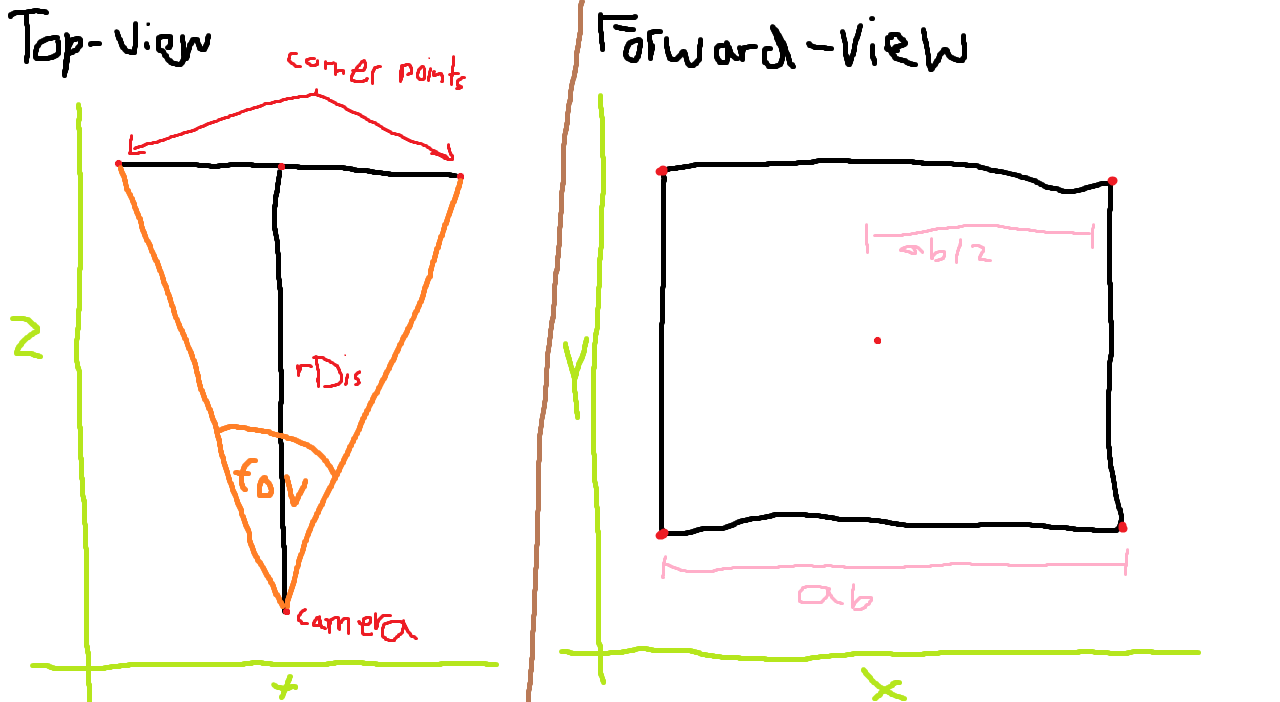# Project 3d coordinates onto 2d screen with angle (Python)

My current project is rendering 3d shapes in a 3d coordinate-system implemented as an array/list. I've got a Camera class with the method snap, witch "takes a photo" of the list from a specific point and renders it in a higher resolution. Here is an example with camera at (100,100,100), the center of the cube at (100,100,120) and the cube size = 15.Currently I am creating a square at `rDis` (=renderDistance) away with the middle point `(camera\[0\], camera\[1\], camera\[3\]+rDis)` and side lengths calculated with the angle `fov`. From there I can just calculate where the corner-points are with some simple math:

``````def getEdgePoints(self):
epm = (self.pos, self.pos, self.pos + self.rDis)        #Gets center of square
ab = round(2*(self.rDis * math.tan(self.fov/2*(math.pi/180))))      #Gets square width
ad = ab #round(2*(self.rDis * math.tan(self.fov/2*(math.pi/180))))  #Just there because I might change the shape from square to a rectangle. Currently the same as ab.

``````

My question is, how would I get these points if there was another angle `an` involved. I'll draw a picture. This is how it's currently:And how It should be with `an`:Note: All corner points are still an equal distance away from the camera. Everything is just rotated `an` degrees.

But with `an` I cant just do `middle point = (camera, camera, camera+rDis)`. How can I calculate that and the corner-points?